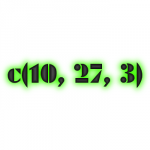Want to share your content on R-bloggers? click here if you have a blog, or here if you don't.Are you an expert R programmer? If so, this is *not* for you. This is a short tutorial for R novices, explaining vectors, a basic R data structure. Here’s an example:

`10 150 30 45 20.3`

And here’s another one:

`-5 -4 -3 -2 -1 0 1 2 3`

still another one:

`"Darth Vader" "Luke Skywalker" "Han Solo"`

and our final example:

`389.3491`

These examples show that a vector is, simply speaking, just a collection of one (fourth example) or more (first and second example) numbers or character strings (third example). In R, a vector is considered a data structure (it’s a very simple data structure, and we’ll cover more complex structures in another tutorial).

# So, how can we set up and use a vector in R?

We can construct a vector from a series of individual elements, using the `c()` function, as follows:

```c(10, 150, 30, 45, 20.3)
```
```##   10.0 150.0  30.0  45.0  20.3
```

(In examples like these, lines starting with `##` show the output from R on the screen).

# Assigning a vector

As you’ll see, once you have entered the vector, R will respond by displaying its elements. In many cases it will be convenient to refer to this vector using a name, instead of having to enter it over and over again. We can accomplish this using the `assign()` function, which is equivalent to the `<-` and `=` operators:

```assign('a', c(10, 150, 30, 45, 20.3))
a <- c(10, 150, 30, 45, 20.3)
a = c(10, 150, 30, 45, 20.3)
```

The second statement (using the <`-` operator) is the most common way of assigning in R, and we’ll therefore use this form rather than the `=` operator or the `assign()` function.

Once we have assigned a vector to a name, we can refer to the vector using this name. For example, if we type a, R will now show the elements of vector a.

```a
```
```##   10.0 150.0  30.0  45.0  20.3
```

Instead of a, we could have chosen any other name, e.g.:

```aVeryLongNameWhichIsCaseSensitive_AndDoesNotContainSpaces <- c(10, 150, 30, 45, 20.3)
```

Strictly speaking, we call this “name” an object.

To familiarize yourself with the vector data structure, now try to construct a couple of vectors in R and assign them to a named object, as in the example above.

To summarize: A vector is a data structure, which can be constructed using the `c()` function, and assigned to a named object using the `<-` operator.

Now, let’s move on to the first set of real exercises on vectors!